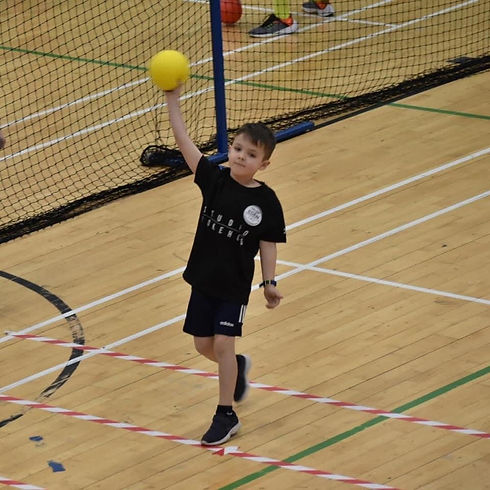# At our Tuesday and Friday sessions we host a Last Player Standing competition. Results from each LPS are added to the table below and winning players are presented with a prize after 5 rounds.## TUESDAY

Mikey = 28 (4 + 9 + 7 + 8)

William = 27 (9 + 8 + 9 + 1)

Hendo = 27 (7 + 7 + 10 + 3)

Jake = 26 (10 + 6 + 10)

Jenson = 26 (5 + 1 + 10 + 10)

Mabel = 19 (1 + 4 + 4 + 10)

Noah = 18 (8 + 10)

Jonah = 18 (6 + 2 + 6 + 4)

Daniel = 15 (2 + 5 + 3 + 5)

Simon = 14 (8 + 6)

Jacob = 14 (3 + 3 + 1 + 7)

Maya = 14 (5 + 9)

Sam = 4 (2 + 2)

## FRIDAY

Noah = 25 (9 + 7 + 9)

Max = 16 (8 + 8)

Hendo = 15 (7 + 4 + 4)

Mabel = 15 (3 + 6 + 6)

Bailey = 14 (4 + 3 + 7)

Seb = 9 (6 + 3)

Jack = 6 (5 + 1)

Maya = 5

Isla = 5

William = 3 (1 + 2)

Will = 2

Theo = 2

Seth = 1